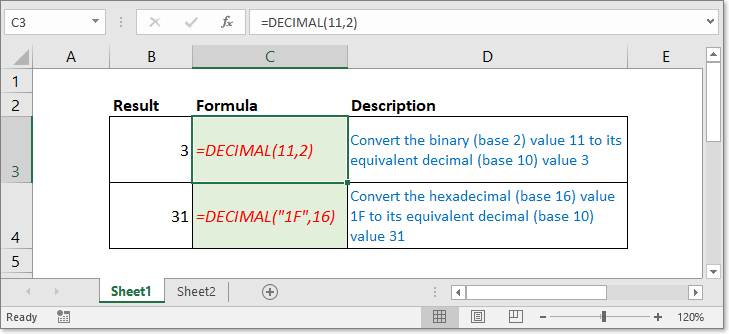Tip: Other languages are Google-Translated. You can visit the English version of this link.orRegisteror

## Excel DECIMAL function### Description

The DECIMAL function converts a text representation of a number in a base into its equivalent decimal number. Take a instance, 11 is a text representation of 3 in base 2, using the formula =DECIMAL(11,2) convert 11 into decimal number 3.

### Syntax and arguments

Formula syntax

Arguments

 Text: Required. The text representation of the number that you want to convert to decimal number. The length of the text must be less than 256. Radix: Required. The base of the supplied number, must be an integer ≥ 2 and ≤ 36.

Return Value

The DECIMAL function returns a decimal number.

Notes:

1. The DECIMAL function is unavailable in the earlier versions of Excel 2013.

2. The Text argument is no case sensitive.

• #NUM! error value: Occurs if either one appears:
• The Radix argument is < 2 or > 36
• The Text argument and Radix argument are not a pair.
• #VALUE! error value: Occurs if either one appears:
• The Text argument is longer than 255 characters
• The Radix argument is nonnumeric value.

### Usage and Examples

Before using the DECIMAL function, you should read below table firstly, which list usually used text representation of numbers and the bases.

 Base Alpha-Numeric Characters Binary (2) 0,1 Octal (8) 0-7 Decimal (10) 0-10 Hexidecimal (16) 0-9 then A-F

Before using the DECIMAL function, you should read below table firstly, which list usually used text representation of numbers and the bases.

 Formula Description Result =DECIMAL(1111,2) Convert the binary (base 2) value 111 to the equivalent decimal (base 10) value 15 =DECIMAL("C2",16) Convert the hexadecimal (base 16) value C2 to its equivalent decimal (base 10) value 194 =DECIMAL(12,8) Convert the octal (base 8) value 12 to the equivalent decimal (base 10) value 10

#### Relative Articles

• Convert decimal to times
This article introduce two different methods to convert decimal number to time format. Take instance, convert decimal hour 31.23 to time format 31:13:48.

### Excel Productivity Tools

Would you like to complete your daily work quickly and perfectly? Kutools for Excel brings 300 powerful advanced features (Combine workbooks, sum by color, split cell contents, convert date, and so on...) and save 70% time.

• Designed for 1500 work scenarios, helps you solve 80% Excel problems.
• Reduce thousands of keyboard and mouse clicks every day, relieve your tired eyes and hands.
• Become an Excel expert in 3 minutes. No longer need to remember any painful formulas and VBA codes.
• 60-day unlimited free trial. 60-day money back guarantee. Free upgrade and support for 2 years.

#### Brings Efficient Tabs to Office (include Excel), Just Like Chrome, Firefox, And New IE

• Office Tab Increases your productivity by 50% when viewing and editing multiple documents.
• Reduce hundreds of mouse clicks for you every day, say goodbye to mouse hand.
• One second to switch between dozens of open documents!Say something here...
symbols left.